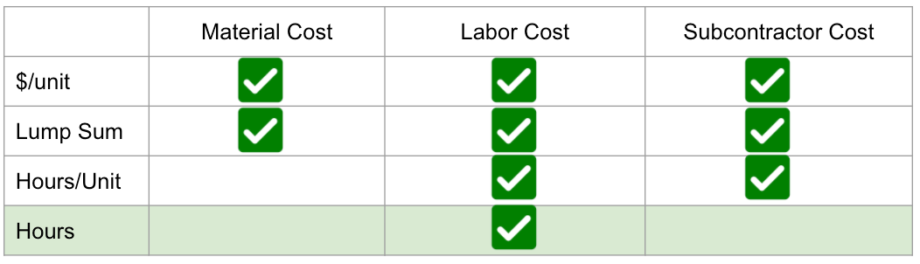# How the Hours Cost Calculation Works

### Overview

The Hours cost calculation is a mix between the Lump Sum and the Hours/Unit calculation. This is great for when you want to charge a customer for a certain number of hours, regardless of the quantity / size of the project.

Like Lump Sum, Hours will ignore the quantity of the part itself. For this calculation, you will simply enter the total hours you want to charge, and the labor rate to use. Clear Estimates will calculate the cost of the part using the labor hours and labor rate!

(Hours * Labor Rate) = Labor Cost

### Where You Can Use It

The hours calculation option is available for your Labor costs only! That means you can set 3 Hours of labor at \$50/hr, and the labor cost will be \$150 regardless of if the part is 10 square feet or 500!

#### What costs can be hours?### Examples

Charge Hours for Labor

Charging your customer a certain number of hours for labor is easy!

Just open the part using the pencil button (far right). Then, make sure the "Labor Cost Calculation" is checked for your part.

Next, select the "Hours" calculation type for labor costs.

Last, simply enter the hours (how long it will take) and the labor rate!